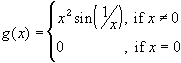#Interactive Real Analysis

Next | Previous | Glossary | Map

## 6.6. A Function Primer

### C(1) FunctionThe function g is continuous on R, differentiable on R, but the derivative is not continuous.

This is the graph of the function. Zoom in by clicking and dragging the mouse, or select Options to change the the power of x. Experiment with different powers. Is the function still differentiable at zero if n = 1 or n = 0 ? If yes, is the derivative continuous ? How about if n > 2 ?